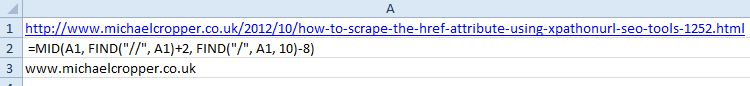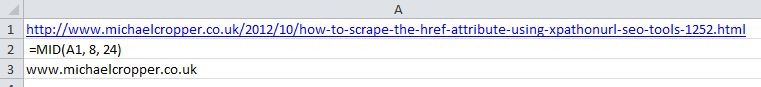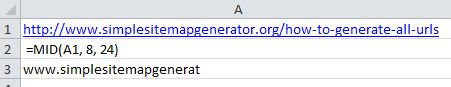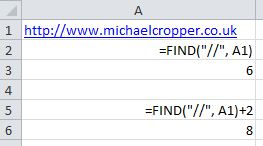Often when analysing backlinks it is important to de-duplicate rows and rows of data into some kind of useful list of domains that you can work with. Often this can be quite a tiresome tasks but with a few Excel formulas it is possible to get the domain name from a long URL.

If you are just looking for the quick answer on how to get the domain name from a cell that contains a URL in Excel then it is;

=MID(A1, FIND(“//”, A1)+2, FIND(“/”, A1, 10)-8)

=MID({CELL OF FULL URL}, FIND(“//”, {CELL OF FULL URL})+2, FIND(“/”, {CELL OF FULL URL}, 10)-8)If you are looking for a bit more of an understanding then read on a little further…

## Explanation of Excel Formula

Lets break down the formula into the key components the finally join them all together into the full formula.

### MID(A1, … , … )

The MID formula in Excel is translated as =MID(text, start_number, number_of_characters) and is designed to create a sub-string of the original string. This can be very handy if you know exactly what position you want to start at and how many characters you want to put into the sub-string, although domain names are different lengths which doesn’t help much for doing this on scale.

If we use the previous blog post as an example to illustrate this then we could use the formula  =MID(A1, 8, 24);While this is great if wanting to get the domain name from lots of URLs on the same domain (although alternatively you could just type the domain out on your keyboard….) – this formula falls short when you are trying to get the domain name from different URLs from different domains as shown below;Half of the domain name is missing…Not very useful…

Fortunately we can group this together with other formulas to figure out where the start position should be and how many characters should be included within the sub-string.

### FIND(“//”, A1)+2

The next bit to the formula is figuring out where to start the sub-string. To do this we use the FIND function which as you may have guessed….finds something. So if we take the example FIND(“//”, A1)then what this formula is doing is finding the first occurrence of the text// within cell A1 – in this case, it is finding where the http:// bit ends. Why add 2? Because the FIND function in Excel identifies the starting point opposed to the end point as in the example below;The 6th character in the domain name in the example above is the first forward slash. So if we used that in combination with the MID formula then we would end up with something like//www.michaelcropper.co.uk being returned which contains the leading slash which isn’t correct. So if we add 2 (as in two characters to the right) then the returned result ends up beingwww.michaelcropper.co.uk instead which is what we are looking for.

### FIND(“/”, A1, 10)-8

The next bit is figuring out the number of characters that should be used in the sub-string. This is using the same FIND function as in the previous step but using an additional argument within the formula (the 10). The FIND function within Excel works as follows: =FIND(sub-string, string, optional_start_position). So in this example, the 10 represents where the FIND function should begin looking for the first instance of the forward slash.

Since you cannot have a forward slash in the domain name itself and the two initial forward slashes within the http:// are at a maximum position of 8 characters along, then the first forward slash after this point has to be the end of the domain name and the start of the URI.

Why -8 characters? Because the full length of http:// is 8 characters and I am not that interested in that for this purpose so I get rid of it. If you want to keep this in then just remove the -8 bit of the formula.

## Joining it all together

So while the initial formula may seem a little daunting at first, when you break it down into its smaller components it is not too difficult to figure out what is going on. If you want to think of the full function as a sentence it can be described as;

=MID(A1, FIND(“//”, A1)+2, FIND(“/”, A1, 10)-8)

Get me the domain name from the full URL which is contained within cell A1. Start the sub-string after the first occurrence of two forward slashes together and end the sub-string at the first occurrence of a forward slash after the first 10 characters.

## Real world example

An example of how this can be used is with competitor backlink analysis to quickly identify all of the unique domains that are linking to your competitors websites but not your own. There will be a blog post to follow on this as it is a little more in-depth for what this post is looking at, but the Excel functions described here about how to get the domain name from a full URL can certainly help speed up that process massively.

The following two tabs change content below.#### Michael Cropper

Founder & Managing Director at Contrado Digital Ltd
Michael founded Contrado Digital in 2013. He has experience working with national and multi-national brands in a wide range of industries, helping them achieve awesome results. Michael regularly speaks at local universities and industry events while keeping up with the latest trends in the digital industry.